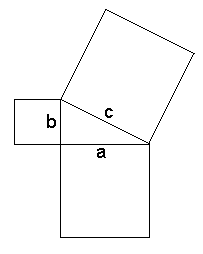Tuesday, January 27

• More Discussion (brief) on the The Sphere  and the Torus.

• How can one distinguish the sphere from a plane (Flatland) based solely on experiences on the surface?
How can one distinguish the sphere from a torus based solely on experiences on the surface?

Use shadows?: look at shadows at the same time of day? This is a "local"  feature of the surface.
Observe "curvature"? This is also a local property.

Circumnavigate (Global)?:  Go West -> return from the East, then  go North-> return from the south.
On a sphere: there will always be 2 points of intersection of the curves determined by the two routes.
On a torus: There will be only one point of intersection of the two routes.
plane: Go West ->  you keep going... there seems to be no return???
Other issues: What about strange gravity? Finding an edge? How do you know when you start?

Comment: How do we know if we continue to walk in Flatland that we could walk forever?
The Halting problem: "Knowing when you start a process, whether or not you will ever stop." This problem is unsolveable for computer programs.

The halting problem is a decision problem which can be informally stated as follows:

Given a description of an algorithm and a description of its initial arguments, determine whether the algorithm, when executed with these arguments, ever halts (the alternative is that it runs forever without halting).

Alan Turing proved in 1936 that there is no general method or algorithm which can solve the halting problem for all possible inputs.

The importance of the halting problem lies in the fact that it was the first problem to be proved undecidable. Subsequently, many other such problems have been described; the typical method of proving a problem to be undecidable is to reduce it to the halting problem.

• Measurement and the Pythagorean Theorem (PT) [1.1]
•a2 + b2 = c2

Show video on PT- Put on reserve in library!
Background: Similar triangles
Area of triangles = 1/2 bh
Area of parallelogram= bh
Scaling: a linear scale change of r gives area change of factor r^2.
3 questions: running, moat, wind power...
Proof of the PT: Similar right triangles c = a^2/c + b^2/c.
applications and other proofs.
Prop. 47 of Euclid.
Dissection Proof.
Prop 31 Book  VI  Similar shapes.
Simple proof of PT using similar triangles of the triangle.
Use in 3 dimensional space.

Puzzles and Polygons [1.2]
• Measuring angles, lengths and areas.
• Squares, rectangles  : 90 degree/ right angle
• parallelograms: opposite angles are congruent, sum of consecutive angles =180 degrees
• triangles  : add to 180 degrees- straight angle [Illustrated physically and with wingeometry]
• Dissections, cut and paste methods of measurement.
• Cutting and reassembling polygons.
• The triangle, quadrilateral, pentagon, and hexagon.
• More on measurements of angles and areas of polygons.
• Activity: 1.2 Ex. 4, 5, 6
• We showed how a quadrilateral can be made from two triangles...
so the sum of its interior angles is 2 * 180 = 360.
• We showed how a hexagon can be made from 4 triangles... so the sum of its interior angles is 4* 180 = 720. If the hexagon has all angles congruent( of equal measurement) then each angle will be 720/6 = 120 degrees!
•  .

• Tangrams. More
• Tangram Activity
Use templates to cut out peices from larger (blue) sheets. [#Partners=3.]

• Cutting and reassembling polygons.